Thermodynamics and Propulsion

16.6 Muddiest Points on Chapter 16

MP 16..1   How do we quantify the contribution of each mode of heat transfer in a given situation?

Developing the insight necessary to address the important parts of a complex situation (such as turbine heat transfer) and downplay (neglect or treat approximately) the other aspects is part of having a solid working knowledge of the fundamentals. This is an important issue, because otherwise every problem will seem very complex. One way to sort out what is important is to make order of magnitude estimates (similar to what we did to answer when the one-dimensional heat transfer approximation was appropriate) to see whether all three modes have to be considered. Sometimes one can rule out one or two modes on the basis of the problem statement. For example if there were a vacuum between the two surfaces in the thermos bottle, we would not have to consider convection, but often the situation is more subtle.

MP 16..2   How specific do we need to be about when the one-dimensional assumption is valid? Is it enough to say thatis small?

The answer really is be specific enough to enable one to have confidence in the analysis or at least some idea of how good the answer is.'' This is a challenge that comes up a great deal. For now, if we say that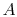is an area defined per unit depth normal to the blackboard then sayingis small, which is a statement involving a non-dimensional parameter, is a reasonable criterion.

MP 16..3   Why is the thermal conductivity of light gases such as helium (monoatomic) or hydrogen (diatomic) much higher than heavier gases such as argon (monoatomic) or nitrogen (diatomic)?

To answer this, we need some basics of the kinetic theory of gases. A reference for this is Castellan, Physical Chemistry, Benjamin/Cummings Publishers, 1986. Two factors contribute, the collision cross section and the average molecular velocity. For the gases mentioned above the dominant factor appears to be the velocity. The kinetic energy per molecule at a given temperature is the same and so the lower the molecular weight the higher the average molecular velocity.

MP 16..4   What do you mean by continuous?

The meaning is similar to the definition you have seen in the math subjects. A way to state it is that the function at a given location has the same value as we approach the location independent of the direction we approach from. To say this in a more physical manner, the temperature as a function of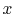has the same value at, say, whether we approach locationfrom the left or from the right. In terms of what the function looks like, it will have no jumps'' or discontinuous (step) changes in value.

MP 16..5   Why is temperature continuous in the composite wall problem? Why is it continuous at the interface between two materials?

We can argue this point by supposingwere not continuous, i.e., there was a different temperature on the two sides of an interface between two materials, with the interface truly a sharp plane. If so, there would be a finite temperature difference across an infinitesimal distance, leading to a very large (infinite in the limit) heat flux which would immediately erase the temperature difference. This argument could also be applied to any location inside a solid of uniform composition, guaranteeing that the temperature is continuous in each material.

MP 16..6   Why is the temperature gradientnot continuous?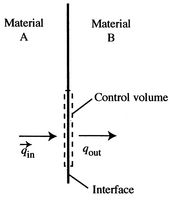As derived in class, across an interface the heat flux is continuous. From the first law, for a thin control volume that encloses the interface the net heat flow into the control volume is zero. As sketched in Figure 16.12, the contribution from the heat flux at the upper and lower ends of the control volume is negligible so the heat flux in one side must be the same as the flux out of the other. The heat flux, however, is related to the temperature gradient by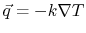, or, for one dimension,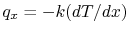. If the heat flux is continuous, and if the thermal conductivity,, is not the same in the two materials, thenis not continuous.

MP 16..7   Why is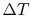the same for the two elements in a parallel thermal circuit? Doesn't the relative area of the bolt to the wood matter?

In terms of the bolt through the wood wall, the approximation made is that the bolt and the wood are both exposed to the same conditions at the two sides of the wall. The relative areas of the bolt and the wood indeed do matter. Suppose we consider a square meter area of wood without bolts. It has a certain heat resistance. If we now add bolts to the wall, the resistance of each bolt is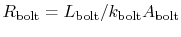. If there are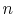bolts, they will be in parallel, and the effective area will betimes the area for one bolt. The thermal resistance of the wood will be increased by a factor of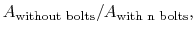which is larger than unity. In summary, the amount by which the heat transfer is increased depends on the fractional area with low thermal resistance compared to the fractional area with high thermal resistance.

UnifiedTP